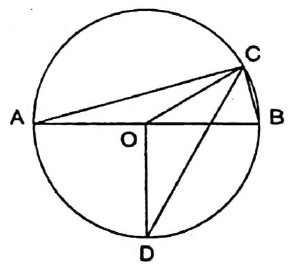# MT #12: Full-syllabus

### Instructions

• You are responsible for keeping time. Email all your solutions by 17:05 Hrs IST.
• Find an email from me with the subject line ‘Mock test 12: Full-syllabus’. Send your solutions (images) as replies to this email.
• Adjust/Reduce the resolution of the camera so that each image is less than 500 KB in size.
• Total marks: 100 (10x4=40 for Part A + 6x10=60 for Part B)

For students who miss the live test (members only)
Self-administer the mock test and email your solutions before 24 July, 23:59 Hrs. Your solutions will be evaluated but marks won’t be counted for official use in the future. Solutions submitted after 24 July, 23:59 Hrs will not be evaluated. As per the rules of CMI entrance exam, no calculators or log tables must be used.

## Part A: Short-answer type questions

Submission file: Write answers to all the ten questions on a single sheet of paper. Email a picture of your answer sheet. Name the file as PartA.jpg or PartA.png.

For this part, answers must be written without any explanation.

1. Find a value $$k$$ such that $$f(x)=x^{2}+kx-17, f(4)=f^{\prime}(4)$$.

2. Suppose we have a number $$x$$ such that: \begin{align*} x & \equiv 1 \pmod{2}\\ x & \equiv 2 \pmod{3}\\ x & \equiv 3 \pmod{5} \end{align*} List all the possible values of $$x \pmod{30}$$.

3. $$AEBCD$$ is a pentagon such that $$ABCD$$ is a rectangle and $$AEB$$ is an isosceles triangle. A circle passes through $$A,B,C,D$$ and $$E$$. This circle has radius 1 unit. Find the value of $$|AD|$$ for which the rectangle and triangle have the same area.

4. If $$a=(\sqrt{3}+\sqrt{2})^{-3}$$ and $$b=(\sqrt{3}-\sqrt{2})^{-3}$$. Find the value of: $\frac{1}{(a+1)}+\frac{1}{(b+1)}$.

5. Find the value of $$n$$ that maximizes$$\left|\sqrt{n^{2}+4 n+5}-\sqrt{n^{2}+2 n+5}\right|$$.

6. Numbers from 1 to 9999 are written on a paper. How many times is the digit 2 written?

7. Suppose $$f: \mathbb{R} \rightarrow \mathbb{R}$$ is an odd and differentiable function. Then for every $$x_{0} \in \mathbb{R}, f^{\prime}\left(-x_{0}\right)$$ is equal to:
(a) $$f^{\prime}\left(x_{0}\right)$$
(b) $$-f^{\prime}\left(x_{0}\right)$$
(c) 0
(d) -1
(e) None of these.

8. How many ways are there to choose positive integers $$x$$ and $$y$$ such that the least common multiple of $$x$$ and $$y$$ is 144 ?
9. Consider tangent circles $$\gamma_{1}$$ and $$\gamma_{2}$$ with centers $$O_{1}, O_{2}$$ and radii $$R, r$$ with $$r < R$$, respectively. Let $$\overline{A B}$$ be a common external tangent of length 16. The area of $$A B O_{1} O_{2}$$ is 160. Find the ordered pair $$(r, R)$$.
10. Let $$a, b \in \mathbb{C}$$ such that $$a+b=a^{2}+b^{2}=\frac{2 \sqrt{3}}{3} i$$. Compute $$|\operatorname{Re}(a)|$$.

## Part B: Subjective questions

Submission files: Each question in this part must be answered on a page of its own. Name the files as B1.jpg, B2.jpg, etc. In case you have multiple files for the same question, say B4, name the corresponding files as B4-1.jpg, B4-2.jpg, etc.

Clearly explain your entire reasoning. No credit will be given without reasoning. Partial solutions may get partial credit.

B1. Consider the quadratic function $$f(x)=x^{2}+b x+c$$ defined on the set of real numbers. Given that the roots of $$f$$ are two distinct prime numbers $$p$$ and $$q$$, and $$f(p-q)=6 p q$$, determine the primes $$p$$ and $$q$$, and the function $$f$$.

B2. $$AB$$ is a diameter of a circle of radius $$10$$ units. $$C$$ is a point on the circle such that the length of arc $$B C=\frac{5 \pi}{3}$$. The bisector of $$\angle A C B$$ cuts the circle at $$D$$. Find the length of $$CD$$.B3. Determine the number of positive integers $$n$$ less than $$10000$$ for which the sum $\frac{1}{2 \times\lfloor\sqrt{1}\rfloor+1}+\frac{1}{2 \times\lfloor\sqrt{2}\rfloor+1}+\frac{1}{2 \times\lfloor\sqrt{3}\rfloor+1}+\cdots+\frac{1}{2 \times\lfloor\sqrt{n}\rfloor+1}$ is an integer.
Notation. $$\lfloor x\rfloor$$ denotes the largest integer that is less than or equal to $$x$$.

B4. We say that a function $$f: \mathbb{R} \rightarrow \mathbb{R}$$ is flippy if $$f(f(x))=-x$$ for all $$x \in \mathbb{R}$$
(a) Prove or disprove: $$f$$ is always injective.$$\;\;\;$$ [2 marks]
(b) Give an example of a flippy function.$$\;\;\;$$ [3 marks]
(c) Prove that there is no flippy function that is continuous. $$\;\;\;$$ [5 marks]

B5. (a) Suppose $$p$$ and $$q$$ are relatively prime numbers. Compute the limit: $\lim_{k\rightarrow \infty} \sqrt{k^{2} q^{2}+2 p k}-\sqrt{k^{2} q^{2}} \;\;\;\;\;\text{[4 marks]}$

(b) Find the value of: $$\displaystyle\lim_{x\rightarrow\infty}\dfrac{1}{x} \int_{0}^{x} \frac{1}{3+\cos t}dt\;\;\;\;\; \text{[6 marks]}$$

B6. A nonnegative integer $$k$$ is special when all pairs of 1s in the binary representation of $$k$$ are separated by at least two zeroes. For example, $$9=1001_{2}$$ is special, but $$10=1010_{2}$$ is not special. How many special numbers are less than $$2^{15}$$?